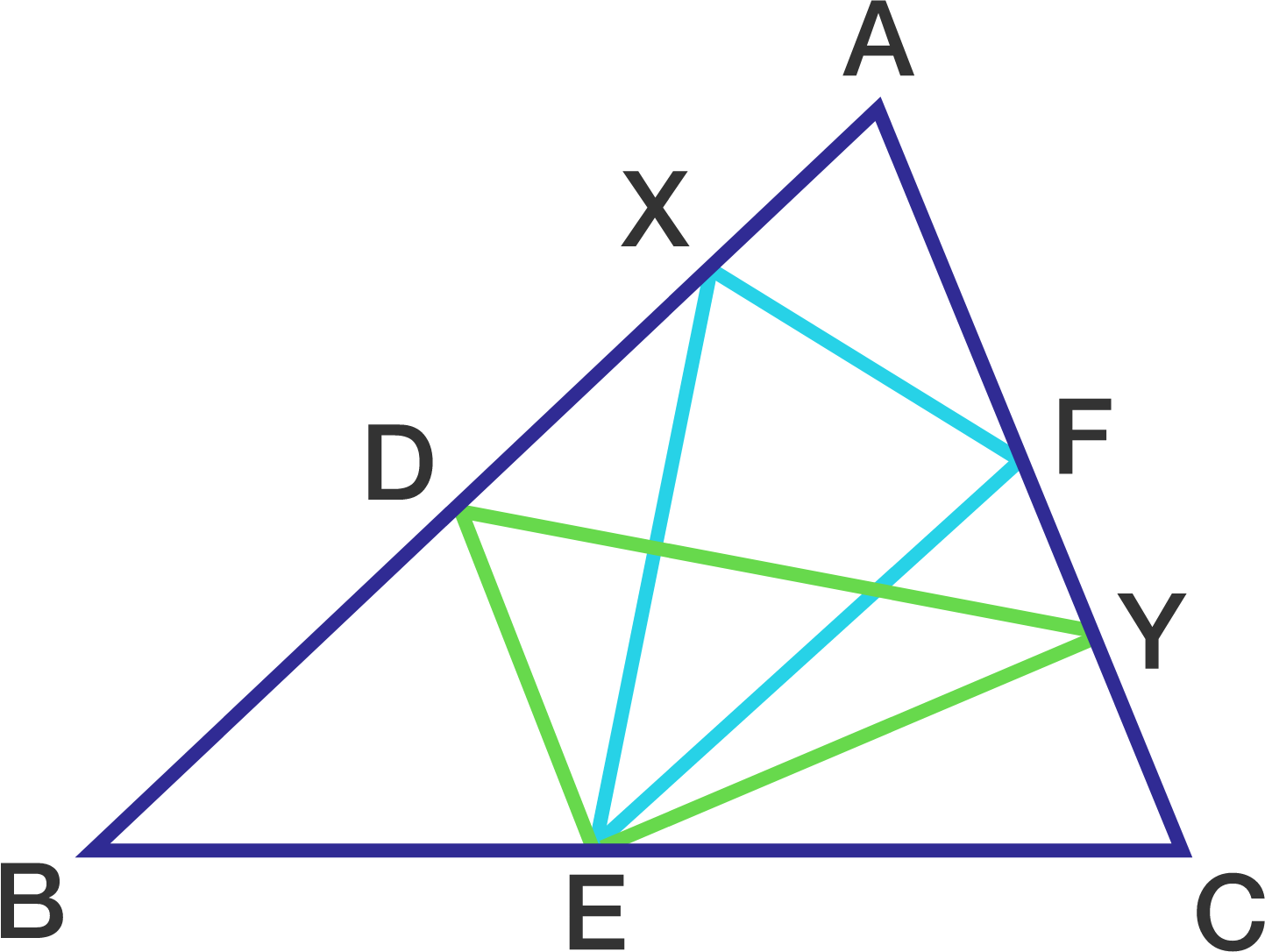# Nasty Triangle

Geometry Level 1The diagram above shows a triangle, it is known that $ADEF$ is a parallelogram, $X$ is a point on line $AB$, $Y$ is a point on line $AC$. If the area of $\triangle XEF$ is 1, then the area of $\triangle DEY$ is...

×